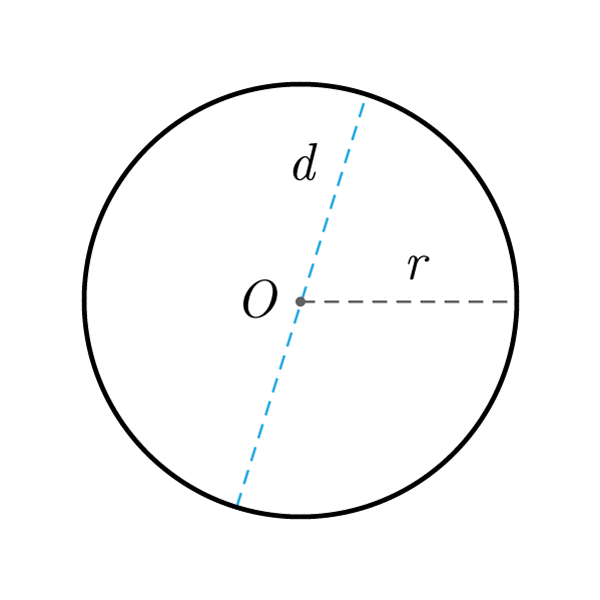# Circle

 Center $$O$$ Radius $$r$$ Diameter $$d$$ Circumference $$C$$
$$C = 2 \pi r$$
Circumference
$$r = \frac{C}{2 \pi }$$
$$A = \pi {r}^2$$
Area
$$r = \sqrt{\frac{A}{\pi}}$$
$$d = 2 r$$
Diameter
$$r = \frac{d}{2}$$

## Definition

A circle is the set of all points in a plane that are equidistant from a given point called the center of the circle.

## Properties

1. Radius: segment that extends from the center of the circle to any point of the circumference
2. Diameter: segment passing through the center that extends two points of the circumference. It's double the radius
3. Pi (symbol $\pi$) constant value approximated as $$\pi \simeq 3.14$$## Circle Formulas

Data Formula
Circumference C = 2 π r
Area A = π r2
Diameter d = 2 × r
Radius r = C / (2 π)
Radius r = √( A / π )
Radius r = d / 2